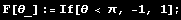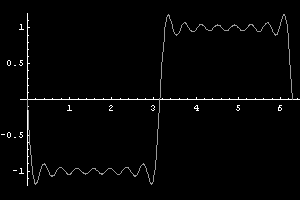# Circular Harmonics

Spherical harmonics can be used in 3d to project lighting environments into a small set of coefficients that compactly represent incoming light from all directions. Ravi Ramamoorthi and Pat Hanrahan introduced this in their excellent paper An Efficient Representation for Irradiance Environment Maps. Folks like Peter Pike Sloan have run with this and used spherical harmonics for encoding occlusion information and other attributes that depend on 3d direction.

Circular harmonics are like the little brother to spherical harmonics and can be used to efficiently encode values that vary based on a single angle instead of two. For example Alex Evans (aka statix) used them in Rag Doll Kung Fu to store the lighting of an object at all angles as a light rotates around it. But just like spherical harmonics any interesting attributes that vary with angle can also be represented in this way.

The circular harmonic basis functions are given by the Fourier series:We can verify that the basis functions are orthogonal by testing all pairswe can also see that they are orthonormalTo decompose some function into a set of circular harmonic coefficients we have to project the function onto each of the basis functions like so:Here wi is the ith weight, F is the function we're projecting, and Bi is the ith circular harmonic function.

Ok, so let's try it out. Here's a simple step function for us to start with:Integrating this function with each circular harmonic basis function gives us the weights:Now if we scale the basis functions by the weights and add them together we get our reconstruction of the original function:Pretty sweet huh? That's a reconstruction using the first 32 circular harmonics. The nice property here is that the evaluation of the reconstructed function is a simple set of multiplications and additions which can be efficiently computed with dot product instructions in pixel and vertex shaders.

Now for your amusement here's the decomposition and reconstruction of some other functions: